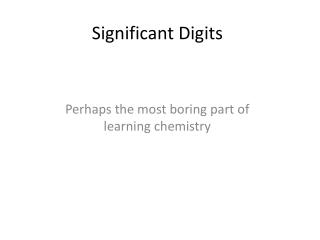DownloadDownload PresentationSignificant Digits

# Significant Digits

Download Presentation## Significant Digits

- - - - - - - - - - - - - - - - - - - - - - - - - - - E N D - - - - - - - - - - - - - - - - - - - - - - - - - - -
##### Presentation Transcript

1. Significant Digits Perhaps the most boring part of learning chemistry

2. Measuring things • It is never possible to measure something exactly • There will always be one number in a measurement that is estimated • The number of digits in a measurement that are known with certainty PLUS the estimated number are called significant digits

3. Whether measuring length… What is this measurement? How many significant digits are there?

4. Whether measuring length… 11.64 cm 4 significant digits

5. Or volume… What is this measurement? How many significant digits are there?

6. Or volume… 6.62 ml Three significant digits

7. Or mass… What is this measurement? How many significant digits are there?

8. Or mass… 373.34 g 5 significant digits

9. Problems occur when we use these measurements in doing calculations… For example, if we were measuring the side of a cube with our ruler, and each side measured 11.64 cm, the volume calculated would be 1577.098944cm3 ! To keep things a bit under control, there are a few rules we must follow…

10. When multiplying or dividing measurements… • The product or quotient can have NO MORE significant digits than the measurement with the least number of significant digits • In our previous example with the cube, 1577.098944cm3 becomes 1577 cm3, with 4 significant digits.

11. When adding or subtracting measurements… • The sum or difference can have NO MORE places after the decimal point than the measurement with the least number of decimal places • If we measured 6.62 ml of liquid with our graduated cylinder, and then 6.6 ml with a cheaper model, the sum of both would be 13.2 ml, with one digit after the decimal place

12. Rounding off…

13. When using a calculator to perform functions involving measurements, always know the number of significant digits the result should have… If the number immediately after the last significant digit is greater than or equal to 5, then round the last significant digit up 125.826 to three significant digits… .826 ≥ .500, therefore round 125 up to 126

14. When using a calculator to perform functions involving measurements, always know the number of significant digits the result should have… If the number immediately after the last significant digit is less than 5, then round the last significant digit down 125.226 to three significant digits… .226 ≤ .500, therefore leave 125 alone

15. Round to the number of significant digits 147.25 two sig digs 147.25 three sig digs 2.055 one sig dig 2.055 two sig digs 2.055 three sig digs 70.1819 one sig dig 70.1819 three sig digs 70.1819 five sig digs

16. More rules… Zeroes in the beginning of a number never count. .000454 3 sig figs

17. More rules… Zeroes at the end of a number count only if there is a written decimal point. 63.00 4 sig figs 6300 2 sig figs

18. More rules… The digits 1 - 9 always count. 146.72 5 sig figs

19. More rules… Zeroes between the digits 1 - 9 always count. 120.45 5 sig figs

20. How many significant digits? 425000 .00063 95007 .10006 700.0 .0000120 80. 1.00012 .003700 756.90 2000 .045 00.0075

21. How to PO your dad • He sends you down to the store to buy some carpet. He says he measured the room to be 9 feet by 7 feet. • You come home with 60 sq. ft. of carpet. • He says, “Are you nuts? Can’t you multiply?” • You tell him that Mr. Stine taught you all about significant digits and rounding in chemistry class, and that he should have measured more accurately.

22. Density lab • We will measure the mass and dimensions of ten different regular solids • We will use Excel to figure the • Volume • Density • Error • Percentage error